Back to Kemp Acoustics HomeNext: Numerical implementation Up: Review of input impedance Previous: The radiation impedance matrix   Contents

## Projecting the impedance matrix

The equations for projection of the impedance matrix were derived in section 2.6. Remember that the labels (0), (1) and (2) refer to planes 0, 1 and 2 in figure 4.1.
By way of summary, the equation for projection across a discontinuity is(4.3)

where
,
are the cross-sectional areas and
(4.4)

The projection matrices are given by(4.5)

where
with
and
.

The equation for projection through a distance
is (N.B. This is a correction to the original version of my thesis and D2 is given in equation (2.41))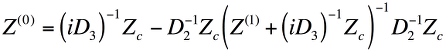(4.6)

where(4.7)

Here(4.8)

is the wavenumber of theth mode along the tube neglecting the effect of losses; the corresponding expression for lossy propagation is given in section 2.4.1.is theth zero of the Bessel function
as tabulated in appendix A.

Back to Kemp Acoustics HomeNext: Numerical implementation Up: Review of input impedance Previous: The radiation impedance matrix   Contents
Jonathan Kemp 2003-03-24